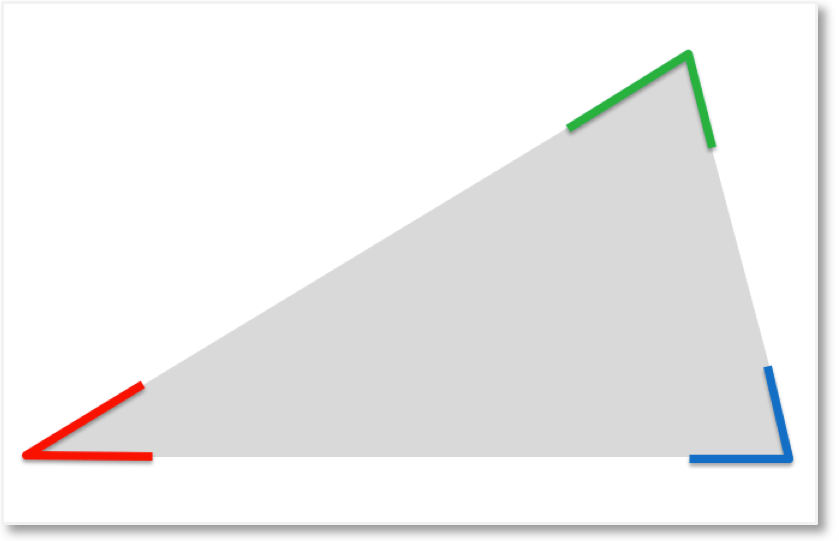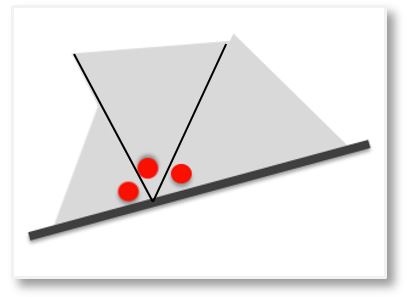# Why do Angles in a Triangle Add Up to 180 Degrees?

Why do Angles in a Triangle Add Up to 180 Degrees?

## Why do Angles in a Triangle Add to 180 Degrees?

To investigate the sum of the angles in a triangle, we can begin by marking each angle with a different colour.We can use this to find out what the angles in a triangle add up to.

If we move these angles so that they fit in next to each other, we can see that they rest on a straight line.Angles on a straight line add up to 180°.

Therefore, angles in a triangle also add up to 180°.

You can test this at home by following these steps:

1) Cut out a triangle2) Mark the outer angles3) Cut these angles off4) Place these marked angles togetherYou should be able to place these angles onto a straight line.Angles in a triangle add up to 180°.error: Content is protected !!